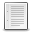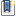File : Details

# One dimensional Time Series to M X N matrix conversion

This function converts a one dimensional time series to an array of M X N matrix given by user
Details
Version
1.0
Author
paawan sharma
Maintainer
paawan sharma
Category
Supported Scilab Version
5.3
Creation Date
April 23, 2015Description
```            //This function converts a one dimensional time series to
//an array of m X n matrix given by user along with original time series x
// Example usage------
//x = floor(100*rand(1,9))
// x = 30.    87.    53.    30.    33.    23.    25.    85.    48.
//w=ts2array(x,4,3)
//Length of Required Matrix is more than original series length
// Hence, 3 zeros will be appended in original time series
// w  =
//    30.    87.    53.
//    30.    33.    23.
//    25.    85.    48.
//    0.     0.     0.
//w=ts2array(x,4,2)
//Length of Required Matrix is less than original series length
// Hence, 1  end data points of series will be lost
// w  =
//    30.    87.
//    53.    30.
//    33.    23.
//    25.    85.              ```Files (1)Miscellaneous file
```
```News (0)Comments (1)Comment from Samuel Gougeon -- May 10, 2015, 01:13:56 AM
```A two-line implementation extended to hypermatrices:

function a = ts2array(ts, s)
dims = [2 1 3:length(s)]
a = permute(matrix(resize_matrix(ts(:),prod(s),1),[s(2) s(1) s(3:\$)]), dims)
endfunction

// TESTS
x  = [ 30.    87.    53.    30.    33.    23.    25.    85.    48.];
ts2array(x,[4 3])
ts2array(x,[4 2])
ts2array(x,[2 4 2])

// RESULTS
-->x  = [ 30.    87.    53.    30.    33.    23.    25.    85.    48.];

-->ts2array(x,[4 3])
ans  =

30.    87.    53.
30.    33.    23.
25.    85.    48.
0.     0.     0.

-->ts2array(x,[4 2])
ans  =

30.    87.
53.    30.
33.    23.
25.    85.

-->ts2array(x,[2 4 2])
ans  =

(:,:,1)

30.    87.    53.    30.
33.    23.    25.    85.
(:,:,2)

48.    0.    0.    0.
0.     0.    0.    0.
```Leave a comment
Login withEmail notifications